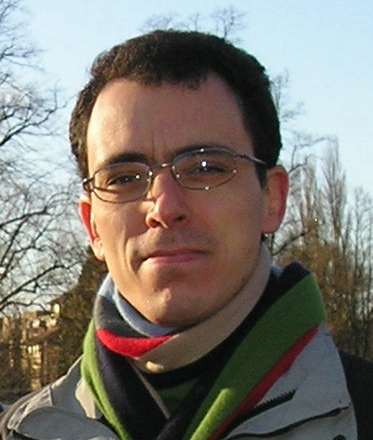# Alfredo Deaño Cabrera

Postdoctoral researcher

Numerical Analysis Group
Department of Applied Mathematics and Theoretical Physics
Centre for Mathematical Sciences
University of Cambridge
Cambridge CB3 0WA
United Kingdom

Telephone: +44 (0)1223 337892

#### Research interests

Gauss and Kummer hypergeometric functions.

Analytical and numerical properties of special functions.

Numerical computation of hypergeometric functions.

Asymptotic analysis.

Papers

1. M. Condon, A. Deaño, A. Iserles, K. Maczynski, T. Xu. On numerical methods for highly oscillatory problems in circuit simulation. DAMTP Tech. Rep. NA2008/12.
2. A. Deaño, N. M. Temme. On modified asymptotic series involving confluent hypergeometric functions. DAMTP Tech. Rep. NA2008/07. To appear in Electronic Transactions on Numerical Analysis.
3. M. Condon, A. Deaño, A. Iserles. On highly oscillatory problems arising in electronic engineering. DAMTP Tech. Rep. NA2008/10. Mathematical Modelling and Numerical Analysis, 43 (2009), 785-804.
4. A. Deaño, D. Huybrechs. Complex Gaussian quadrature of oscillatory integrals. DAMTP Tech. Rep. 2008_04. Numer. Math. 112, 2 (2009), 197-219.
5. A. Deaño, J. Segura, N. M. Temme. Computational properties of three-term recurrence relations for Kummer functions. To appear in J. Comp. Appl. Math.
6. A. Deaño, J. Segura, N. M. Temme. Identifying minimal and dominant solutions for Kummer recursions. Mathematics of Computation, 77 (2008), 2277-2293.
7. A. Deaño, J. Segura. Global Sturm inequalities for the real zeros of the solutions of the Gauss hypergeometric equation. Journal of Approximation Theory, 148, 1 (2007), 92-110.
8. A. Deaño, J. Segura. Transitory minimal solutions of hypergeometric recursions and pseudoconvergence of associated continued fractions. Mathematics of Computation, 76, 258 (2007), 879-901.
9. A. Deaño, A. Gil, J. Segura. Computation of the real zeros of the Kummer function M(a;c;x). Lecture Notes in Computer Science, 4151 (2006), 296-307.
10. A. Deaño, A. Gil, J. Segura. New inequalities from classical Sturm theorems. Journal of Approximation Theory, 131 (2004), 208-230.

Submitted papers

1. M. Condon, A. Deaño, A. Iserles. On asymptotic-numerical solvers for systems of differential equations with highly oscillatory forcing terms.
2. A. Deaño, N. M. Temme. Analytical and Numerical Aspects of a Generalization of the Complementary Error Function. DAMTP Tech. Rep. NA2009/02.
3. M. I. Bueno, A. Deaño, E. Tavernetti. A new algorithm for computing the Geronimus transformation with large shifts. To appear in Numerical Algorithms.

PhD Thesis

Qualitative and numerical aspects of the hypergeometric equation (summary in English).

Curriculum vitae

CV (in English)

CV (in Spanish)

Other

Music. Advanced Certificate (speciality flute, with distinction), ABRSM.

Second flute/piccolo in CUCO (Cambridge University Chamber Orchestra).